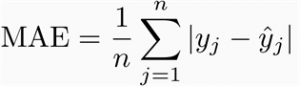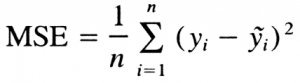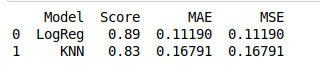Related Articles
ML | Models Score and Error
• Last Updated : 02 Aug, 2019

In Machine Learning one of the main task is to model the data and predict the output using various Classification and Regression Algorithms. But since there are so many Algorithms, it is really difficult to choose the one for predicting the final data. So we need to compare our models and choose the one with the highest accuracy.

Using the sklearn library we can find out the scores of our ML Model and thus choose the algorithm with a higher score to predict our output. Another good way is to calculate errors such as mean absolute error and mean squared error and try to minimize them to better our models.

Mean Absolute Error(MAE): It is the mean of all absolute errorMean Squared Error (MSE) It is the mean of square of all errors.Here, we are using Titanic dataset as our input for Classification problem and modelling our data with Logistic Regression and KNN only. Although, you can also model with other algorithms. You can find the dataset here

 `# importing libraries``import` `numpy as np``import` `sklearn``from` `sklearn ``import` `metrics``import` `pandas as pd`` ` `from` `sklearn.model_selection ``import` `train_test_split``from` `sklearn.linear_model ``import` `LogisticRegression``from` `sklearn.neighbors ``import` `KNeighborsClassifier`` ` ` ` `data ``=` `pd.read_csv(``"gfg_data"``)`` ` `x ``=` `data[[``'Pclass'``, ``'Sex'``, ``'Age'``, ``'Parch'``, ``'Embarked'``, ``'Fare'``,``               ``'Has_Cabin'``, ``'FamilySize'``, ``'title'``, ``'IsAlone'``]]`` ` `y ``=` `data[[``'Survived'``]]`` ` `X_train, X_test, Y_train, Y_test ``=` `train_test_split(x, y,``test_size ``=` `0.3``, random_state ``=` `None``)`` ` `# logistic Regression``lr ``=` `LogisticRegression()``lr.fit(X_train, Y_train)`` ` `Y_pred ``=` `lr.predict(X_test)`` ` `LogReg ``=` `round``(lr.score(X_test, Y_test), ``2``)`` ` `mae_lr ``=` `round``(metrics.mean_absolute_error(Y_test, Y_pred), ``4``)``mse_lr ``=` `round``(metrics.mean_squared_error(Y_test, Y_pred), ``4``)`` ` `# KNN``knn ``=` `KNeighborsClassifier(n_neighbors ``=` `2``)``knn.fit(X_train, Y_train)`` ` `Y_pred ``=` `knn.predict(X_test)`` ` `KNN ``=` `round``(knn.score(X_test, Y_test), ``2``)`` ` `mae_knn ``=` `metrics.mean_absolute_error(Y_test, Y_pred)``mse_knn ``=` `metrics.mean_squared_error(Y_test, Y_pred)`` ` ` ` `compare_models ``=` `pd.DataFrame(``    ``{  ``'Model'` `: [``'LogReg'``, ``'KNN'``],``       ``'Score'` `: [LogReg, KNN],``        ``'MAE'`  `: [mae_lr, mae_knn],``        ``'MSE'`  `: [mse_lr, mse_knn]``    ``})`` ` `print``(compare_models)`

Output:We can now see the score and error of our models and compare them. Score of Logistic Regression is greater then KNN and error is also less. Thus, Logistic Regression will be the right choice for our model.My Personal Notes arrow_drop_up